#### How To Calculate A Variance Estimator in Excel

Variance refers to how widely data sets are scattered around their mean value. We can estimate the variance from a sample of data or from the entire population, all the data.

First, you need to know how to calculate variance in Excel.

Next, you should choose the relevant Excel function to know whether the data is:

• A sample of all the data
• All the data or population
• Numbers only
• Logical values, text, arrays

For the purposes of this guide, test data has been created in document Variance Estimator Sample Data.

## Creating a Variance Estimation of a population – VAR.P

In this scenario a business owner wants to estimate the variance for the annual review ratings for all their employees. So, a population variance estimation is needed.

1. Open Variance Estimator Sample Data, click the VAR.P tab.
2. Click where you would like Excel to display the results, use E6 for this example.
3. In E6, type =VAR.P(
4. Select the ratings – B6 toB12, press Enter, the variance estimate appears in E6.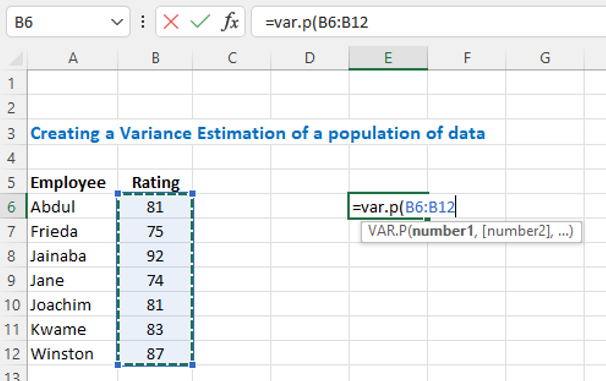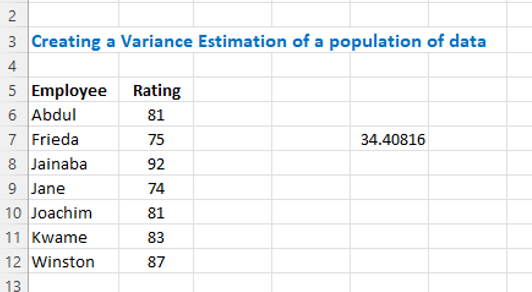## Creating a Variance Estimation of a sample – VAR.S

In this scenario the business owner wants to estimate the variance for the annual review ratings for just the managers. So, we will use the Sample function to estimate the variance.

1. Go to the VAR.S tab.
2. Click where you would like Excel to display the results, use E6 for this example.
3. In E6, type =VAR.S(
4. Select the manager ratings – C10 to C12, press Enter, Excel adds the end bracket and the variance estimate appears in E6.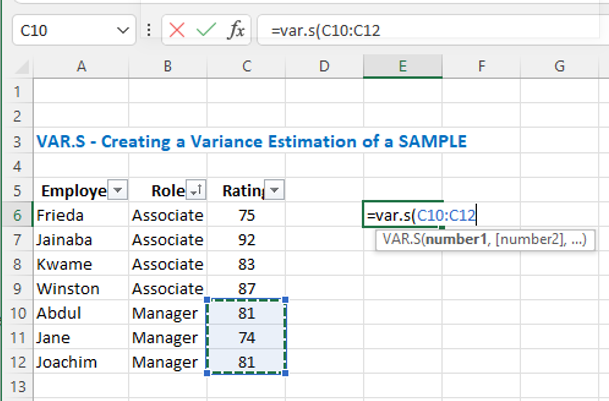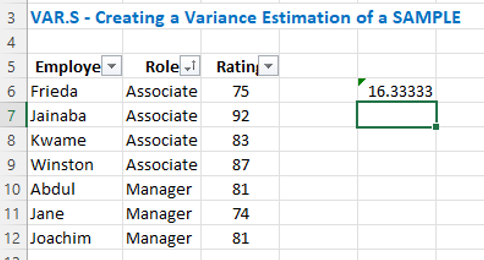If your data contains text or logical values, then use the VARA function for sample and VARPA for population data. Where text and logical values are present in data, they are treated as follows:

• Text is given a value of 0.
• TRUE is evaluated as 1.
• FALSE is evaluated as 0.

## Creating a Variance Estimation of a population with text in the data

In this scenario the business owner wants to estimate the variance for the annual review ratings for all employees. Kwame was on vacation during the review period, as there is text in his rating field, we will use the population VARPA function to estimate the variance.

1. Go to the VARPA tab, note that n/a is listed in the ratings column for Kwame.
2. Click where you would like Excel to display the results, use E6 for this example.
3. In E6, type =VARPA(
4. Select the ratings – C6 to C12, press Enter, the variance estimate appears in E6.
5. Note, the estimated variance is high due to the larger distance between Kwame’s rating and the average (mean) of the combined data.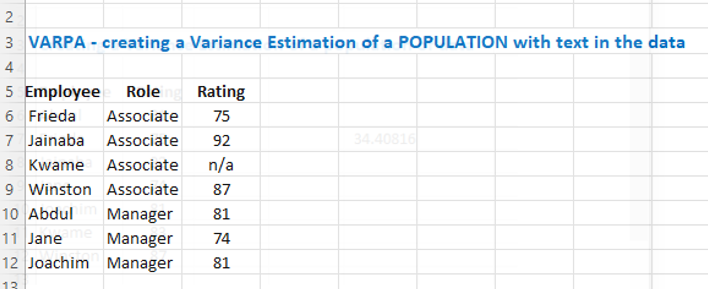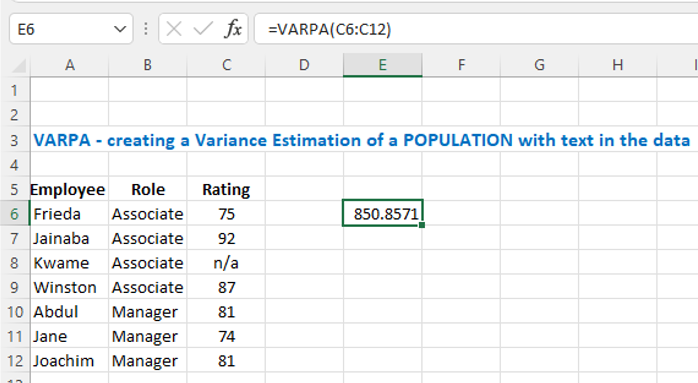Understanding how to calculate a variance estimator is an important part of statistical analysis, as it can help to provide insight into the variability of a dataset and inform decision-making processes in a variety of fields.